# ARCH Test Explained

In time series and econometric analysis, summary statistics and residual diagnosis often lead us to use a somewhat mystifying test known as the Auto-Regressive Conditional Heteroskedasticity (ARCH) effect test, or ARCH test for short. Why exactly do we use the ARCH test?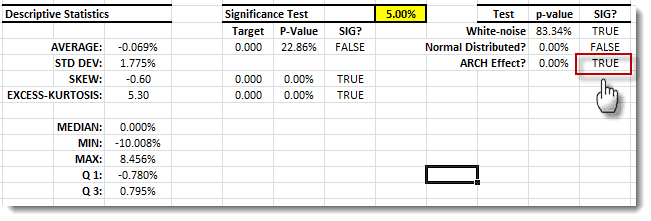You might think of an ARCH test simply as a means of detecting the presence of fat tails in the underlying distribution; but if that were true, what would the test tell us that a test for excess kurtosis could not?

We need to answer some basic questions about the ARCH test: What does it do? How is it related to the white-noise test? When should we utilize what it has to tell us?

Note:

For illustration, we are using the IBM stock price data set for the period between May 17th, 1961, and Nov 2nd, 1962 (Series B in Box, Jenkins, and Reinsell Time series forecast textbook (1976)).

## Background

Let’s assume we have a data set of a univariate, and we wish to determine whether it exhibits an ARCH effect.

1. Construct a new time series, such that:
2. $$y_{t}=x_{t}^{2}$$
3. Form a portmanteau type of test for:
4. $$H_{0}:\rho _{1}=\rho_{2}=...=\rho_{m}=0$$ $$H_{1}:\exists\rho_{k}\neq 0$$

Where

• $H_{0}=$ null hypothesis
• $H_{1}=$ alternative hypothesis
• $m=$ maximum number of lags included in the test
• $\rho_{i}$ the population auto correlation function of the squared time series $(y_{t})$

$1\leq k\leq m$

In essence, the ARCH effect test is a white-noise test, but for the squared time series. In other words, we are investigating a higher order (non-linear) of auto correlation. How can this information be useful?

The ARCH effect has its roots in time varying conditional volatility, so:

$${\sigma _{t}^{2}}=E\left [ {(x_{t}-\bar{x}_{t})}^{2} \right ]=E\left [ x_{t}^2 \right ]-\bar{x}_{t}^{2}$$

Where

• $\sigma _{t}^2=$ conditional variance
• $\bar{x}_{t}=$ conditional mean

Assuming the time series does not have a significant mean (typical in financial time series), then the conditional variance is expressed as:

$$\sigma_t^2=E\left [ (x_t-\bar{x}_t)^{2} \right ]=E\left [ x_t^2 \right ]=E\left [ y_t \right ]\approx x_t^2$$

Assuming the squared time series $(y_t)$ is serially correlated, then conditional volatility $(\sigma_t)$ varies over time and exhibits a clustering phenomenon (e.g. periods of swings followed by periods of relative calm).

In sum, the ARCH test helps us to detect a time-varying phenomenon in the conditional volatility and thus suggests different types of models (e.g. ARCH/GARCH) to capture these dynamics.

• White-noise test $\rightarrow$ conditional mean $\rightarrow$ ARMA/ARIMA
• ARCH test $\rightarrow$ conditional volatility $\rightarrow$ ARCH/GARCH

Q: Can we have a significant serial correlation in the original time series and a serial correlation in the squared time series? If so, how can we model that?

A: Yes; we use an ARMA-GARCH mixture model.

## Analysis

Let’s take a close look at the logarithmic daily returns time series: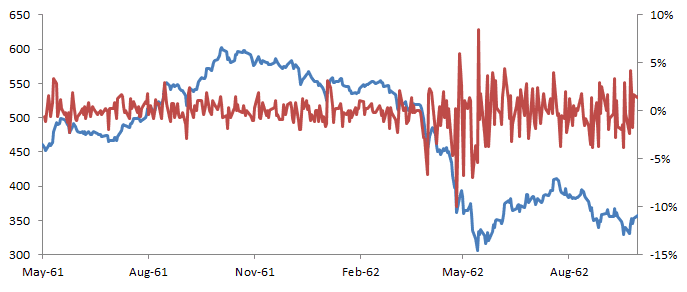The daily return plot suggests a stationary process with zero mean, but the volatility exhibits periods of relative calm followed by swings (aka volatility clustering).

The white-noise test identifies insignificant serial correlation in the time series, but the ARCH effect is significant and indicative of time-varying volatility.

### 1. Daily Returns Distribution

Let’s construct the empirical distribution of the sample time series, and examine the tails in comparison with those of a Gaussian distribution.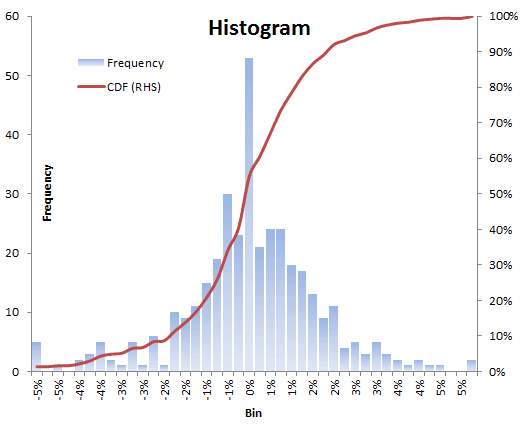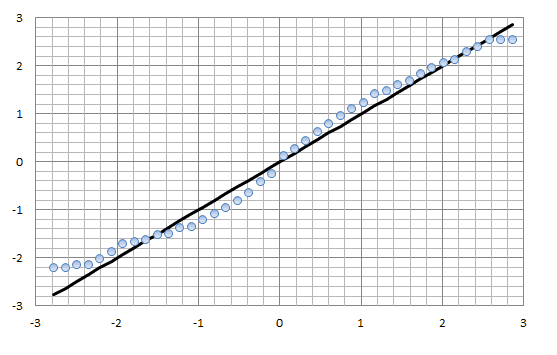The Q-Q plot shows an asymmetrical view of the distribution tails; the distribution’s left tail (i.e. extreme negative returns) are far more deviant from what the Gaussian distribution suggests. This is a well-documented phenomenon in the financial time series.

### 2. Correlogram Analysis

By now, we’ve established that the logarithmic daily returns distribution has fat tails (and maybe fatter on the left side than on the right), but where is the time-varying claim coming from?

In the descriptive statistics table, the ARCH effect suggests a significant serial correlation in the squared time series. Let’s do the following:

1. Construct the squared time series $(y_t)$.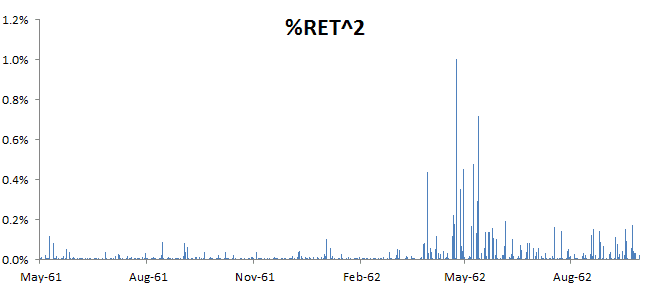2. Run NumXL descriptive statistic wizard, and generate the summary table. The white noise test on the squared time series is equivalent to the ARCH test on the original time series.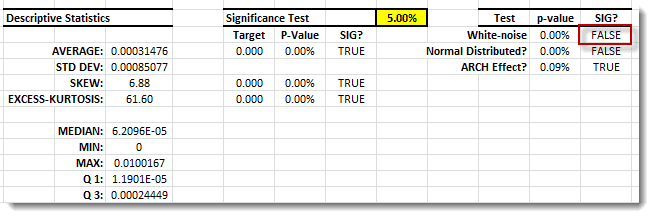Notice:

The ARCH effect for the squared time series is significant, so does this mean we have a time-varying kurtosis (fourth moment)? Let’s keep this question in mind, and revisit it in a separate tutorial!

3. Using the NumXL Correlogram wizard, generate the ACF/PACF table and plot for the squared time series.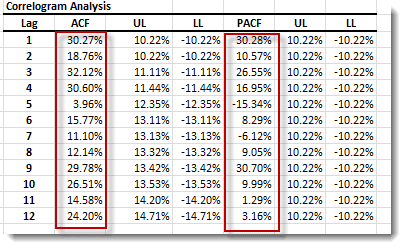The ACF/PACF Plot for the squared time series is shown below: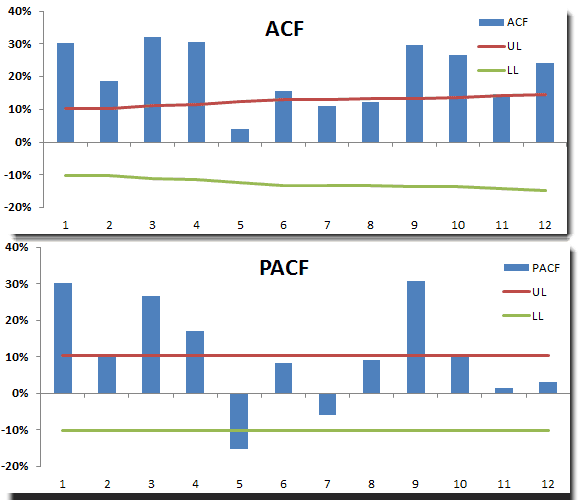### 3. ARCH Modeling

Using the ACF/PACF tables/plot, we can proceed to model the conditional volatility as an ARMA model (aka GARCH). As in ARMA, we need to identify the AR and MA order of the conditional volatility model.

## Conclusion

The ARCH test is a vital tool for examining the time dynamics of the second moments (i.e. conditional variance). The presence of a significant excess kurtosis is not indicative of time-varying volatility, but the reverse is true: a significant ARCH effect identifies time-varying conditional volatility, volatility clustering (or mean reversion), and, as a result, the presence of a fat-tailed distribution (i.e. excess kurtosis > 0).

The ARCH test is a vital tool for examining the time dynamics of the second moments (i.e. conditional variance). The presence of a significant excess kurtosis is not indicative of time-varying volatility, but the reverse is true: a significant ARCH effect identifies time-varying conditional volatility, volatility clustering (or mean reversion), and, as a result, the presence of a fat-tailed distribution (i.e. excess kurtosis > 0).

Finally, notice that for financial time series, the negative returns deviate more from normality than positive ones.